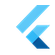Flutter Engineflutter::Point Class Reference

`#include <test_codec_extensions.h>`

## Public Member Functions

Point (int x, int y)

~Point ()=default

int x () const

int y () const

bool operator== (const Point &other) const

Point ()=default

Point (double x, double y)

Point (const Point &point)=default

Pointoperator= (const Point &other)=default

double x () const

double y () const

bool operator== (const Point &other) const

## Detailed Description

Definition at line 14 of file test_codec_extensions.h.

## ◆ Point() [1/4]

 flutter::Point::Point ( int x, int y )
inline

Definition at line 16 of file test_codec_extensions.h.

References ~Point().

16 : x_(x), y_(y) {}

## ◆ ~Point()

 flutter::Point::~Point ( )
default

Referenced by Point().

## ◆ Point() [2/4]

 flutter::Point::Point ( )
default

Referenced by Point().

## ◆ Point() [3/4]

 flutter::Point::Point ( double x, double y )
inline

Definition at line 16 of file geometry.h.

References operator=(), and Point().

16 : x_(x), y_(y) {}

## ◆ Point() [4/4]

 flutter::Point::Point ( const Point & point )
default

## ◆ operator=()

 Point& flutter::Point::operator= ( const Point & other )
default

Referenced by Point(), and flutter::Size::Size().

## ◆ operator==() [1/2]

 bool flutter::Point::operator== ( const Point & other ) const
inline

Definition at line 22 of file test_codec_extensions.h.

22  {
23  return x_ == other.x_ && y_ == other.y_;
24  }

## ◆ operator==() [2/2]

 bool flutter::Point::operator== ( const Point & other ) const
inline

Definition at line 23 of file geometry.h.

23  {
24  return x_ == other.x_ && y_ == other.y_;
25  }

## ◆ x() [1/2]

 int flutter::Point::x ( ) const
inline

Definition at line 19 of file test_codec_extensions.h.

Referenced by flutter::TEST(), and flutter::PointExtensionSerializer::WriteValue().

19 { return x_; }

## ◆ x() [2/2]

 double flutter::Point::x ( ) const
inline

Definition at line 20 of file geometry.h.

20 { return x_; }

## ◆ y() [1/2]

 int flutter::Point::y ( ) const
inline

Definition at line 20 of file test_codec_extensions.h.

Referenced by flutter::TEST(), and flutter::PointExtensionSerializer::WriteValue().

20 { return y_; }

## ◆ y() [2/2]

 double flutter::Point::y ( ) const
inline

Definition at line 21 of file geometry.h.

21 { return y_; }

The documentation for this class was generated from the following files: